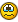# Array of mouseclicks isn't working as expected

## Recommended Posts

OK so I'm going to run this by you guys as I'm pulling my hair outI have a website which is a simple grid and want to click each item on the grid which will run a report.

The code I have is to create an Array for the mouse coordinates

```Global \$ArrayForX

\$ArrayForX = 141
\$ArrayForX = 141 + 202
\$ArrayForX = 141 + 202 + 202
\$ArrayForX = 141 + 202 + 202 +202
\$ArrayForX = 141 + 202 + 202 +202  + 202+ 202
\$ArrayForX = 141 + 202 + 202 +202  + 202+ 202+ 202
\$ArrayForX = 141 + 202 + 202 +202  + 202+ 202+ 202+ 202
\$ArrayForX = 141 + 202 + 202 +202  + 202+ 202+ 202+ 202+ 202
\$ArrayForX = 141 + 202 + 202 +202  + 202+ 202+ 202+ 202+ 202+ 202

Global \$ArrayForY

\$ArrayForY  = 330
\$ArrayForY  = 330+ 76
\$ArrayForY  = 330+ 76 + 76
\$ArrayForY  = 330+ 76 + 76 + 76
\$ArrayForY  = 330+ 76 + 76 + 76 + 76
\$ArrayForY  = 330+ 76 + 76 + 76 + 76 + 76
\$ArrayForY  = 330+ 76 + 76 + 76 + 76 + 76 + 76
\$ArrayForY  = 330+ 76 + 76 + 76 + 76 + 76 + 76 + 76
\$ArrayForY  = 330+ 76 + 76 + 76 + 76 + 76 + 76 + 76 + 76
\$ArrayForY  = 330+ 76 + 76 + 76 + 76 + 76 + 76 + 76 + 76 + 76```

I then created a function  to click the grid

```Func Trend_Pack()
OpenIE("http://rptdev01az/Reports/browse/")

MouseClick('primary', \$ArrayForX[\$X],\$ArrayForY[\$Y] 1)
EndFunc```

Then in my "main" sheet I run it like this

```\$Y = 0
\$X = 0

While \$Y <= 9
Trend_Pack()
\$X = \$X + 1
if \$X = 8 Then
\$Y + 1
\$X = 0
EndIf

WEnd```

Basically at this point it is going through the X axis (clicking each link along the X axis) however when it gets to 9 the \$Y doesn't seem to increment so it keeps going along the same X axis

Edited by CaptainBeardsEyesBeard
##### Share on other sites

There's a much better way of doing this which doesn't require the gymnastics you're going through.

```For \$Y = 0 To 9
For \$X = 0 To 8
Trend_Pack((\$X * 202) + 141, (\$Y * 76) + 330)
Next
Next
Func Trend_Pack(\$Xin, \$Yin)
OpenIE("http://rptdev01az/Reports/browse/")
MouseClick('primary', \$Xin, \$Yin, 1)
EndFunc   ;==>Trend_Pack```

If I posted any code, assume that code was written using the latest release version unless stated otherwise. Also, if it doesn't work on XP I can't help with that because I don't have access to XP, and I'm not going to.
Give a programmer the correct code and he can do his work for a day. Teach a programmer to debug and he can do his work for a lifetime - by Chirag Gude
How to ask questions the smart way!

I hereby grant any person the right to use any code I post, that I am the original author of, on the autoitscript.com forums, unless I've specifically stated otherwise in the code or the thread post. If you do use my code all I ask, as a courtesy, is to make note of where you got it from.

Back up and restore Windows user files _Array.au3 - Modified array functions that include support for 2D arrays.  -  ColorChooser - An add-on for SciTE that pops up a color dialog so you can select and paste a color code into a script.  -  Customizable Splashscreen GUI w/Progress Bar - Create a custom "splash screen" GUI with a progress bar and custom label.  -  _FileGetProperty - Retrieve the properties of a file  -  SciTE Toolbar - A toolbar demo for use with the SciTE editor  -  GUIRegisterMsg demo - Demo script to show how to use the Windows messages to interact with controls and your GUI.  -   Latin Square password generator

##### Share on other sites

Use _ieaction with click, and precisely click your object rather than using mouse coordinates

Edited by jdelaney
IEbyXPATH-Grab IE DOM objects by XPATH IEscriptRecord-Makings of an IE script recorder ExcelFromXML-Create Excel docs without excel installed GetAllWindowControls-Output all control data on a given window.
##### Share on other sites

Wow that solution is so nice. Thank you!

## Create an account

Register a new account

• ### Recently Browsing   0 members

×

• Wiki

• Back

• #### Beta

• Git
• FAQ
• Our Picks
×
• Create New...